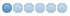# How to read hal pin from embedded panel from an other component

16 Mar 2021 18:15 #202493
This might be a very complex question or just a very simple one.

I want to build a universal debug panel as embedded tab. As the panel should be universal and should be able to show also hal pin from other components, I need to get the component hal pin.

If I do this code in the py file of the embedded tab called "debug_panel", embedded in gmoccapy:
```print(hal.component_exists("gmoccapy"))
print("gmoccapy = ", hal_glib.GComponent("gmoccapy"))
print(hal.component_exists("debug_panel"))
print("timer = ", hal_glib.GComponent("debug_panel"))
print(hal.component_exists("abs"))
print("abs = ", hal_glib.GComponent("abs"))
print(hal.component_exists("motion"))
print("motion = ", hal_glib.GComponent("motion"))```

I get True for gmoccapy, debug_panel, abs and False for motion.
The strange is, that I get for all components the same ID, something like this:
```True
('gmoccapy = ', <hal_glib.GComponent instance at 0x7f07ac079200>)
True
('debug_panel = ', <hal_glib.GComponent instance at 0x7f07ac079200>)
True
('abs = ', <hal_glib.GComponent instance at 0x7f07ac079200>)
False
('motion = ', <hal_glib.GComponent instance at 0x7f07ac079200>)```

If I try to read a PIN from gmoccapy as gmoccapy.blockheigt like this:
`hal_glib.GComponent("gmoccapy")["blockheight"]`
I get an error like:
```Traceback (most recent call last):
File "./timer.py", line 123, in _periodic2
print("gmoccapy = ", hal_glib.GComponent("gmoccapy")["blockheight"])
File "/home/norbert/linuxcnc-org/lib/python/hal_glib.py", line 77, in __getitem__
def __getitem__(self, k): return self.comp[k]
TypeError: string indices must be integers, not str```
If I try a integer, I just get the corresponding letter of the component name.

Is there a way to read a hal pin from an component outside the own component?
A signal would also be OK, but first I will need to get the "component address" and all my test have failed till now.

Norbert

18 Mar 2021 01:29 #202695 by andypugh

I get True for gmoccapy, debug_panel, abs and False for motion.

The component name for motion is "motmod"
```halcmd: show comp
ID      Type  Name                                            PID   State
...
...```

Is there a way to read a hal pin from an component outside the own component?

```import hal
...
v = hal.get_value("motion.program-line")```

I don't know if the hal python interface is documented anywhere but here:
linuxcnc.org/docs/2.8/html/hal/halmodule.html

• 5G-antry
• Offline
• New Member
•11 Jan 2022 19:16 - 11 Jan 2022 19:21 #231473 by 5G-antry
Hello,

Did you manage to read the values of PINs and/or Signals and use them in external software in "real time" (miliseconds range)?
I am currently developing kind of a digital twin of a cnc machine and I am struggeling with this topic.

Best Regards
Last edit: 11 Jan 2022 19:21 by 5G-antry.

13 Jan 2022 02:27 #231625 by Grotius
Hi,

Here is a c++ example.
1. It finds a component by name.
2. It can retrieve a value of a component item.
3. It shows how to retrieve the servo-time
```double hal_interface::print_servo_thread_time(){

/*
if (halpr_find_comp_by_name("matrix") != 0) {
std::cout << "matrix found!" << std::endl;
}
if (halpr_find_param_by_name("matrix.tmax") != 0) {
std::cout << "matrix.time found!" << std::endl;
}*/

//! Get parameter value example
/*
hal_param_t *param;
hal_type_t type;
void *d_ptr;

param = halpr_find_param_by_name("matrix.tmax");
if (param) {
type = param->type;
d_ptr = SHMPTR(param->data_ptr);
static char buf;
snprintf(buf, 14, "%ld", (long)*((hal_s32_t *) d_ptr));

std::cout<<"value:"<<buf<<std::endl;
}*/

//! Get pin value example
/*
if (halpr_find_pin_by_name("matrix.time") != 0) {
std::cout << "matrix.time found!" << std::endl;
} */

hal_data_u *value;
hal_type_t type;
bool *con = nullptr;
int r=hal_get_pin_value_by_name("matrix.mainfunction.time",&type,&value,con);
if(r!=0){
std::cout<<"error from /libhal/hal_interface function"<<std::endl;
}
// std::cout << "matrix.time function result:"<<ok<< std::endl;
// std::cout << "matrix.time function result:"<<value->s<< std::endl;

return value->s;
}```

The following user(s) said Thank You: 5G-antry

13 Jan 2022 02:38 - 13 Jan 2022 02:40 #231626 by Grotius
For example c++:    //! Get pin value example
if (halpr_find_pin_by_name("matrix.time") != 0) {
std::cout << "matrix.time found!" << std::endl;
}

github.com/LinuxCNC/linuxcnc/blob/a2f1fd...src/hal/halmodule.cc
Python line 991 :
#  Get a Pin, Param or signal value
PyObject *get_value(PyObject *self, PyObject *args)

Last edit: 13 Jan 2022 02:40 by Grotius.
The following user(s) said Thank You: 5G-antry